Mutually Exclusive EventsCustom SearchMUTUALLY EXCLUSIVE EVENTS Two or more events are called mutually exclusive if the occurrence of any one of them precludes the occurrence of any of the others. The probability of occurrence of two or more mutually exclusive events is the sum of the probabilities of the individual events. Sometimes when one event has occurred, the probability of another event is excluded (referring to the same given occasion or trial). For example, throwing a die once can yield a 5 or 6, but not both, in the same toss. The probability that either a 5 or 6 occurs is the sum of their individual probabilities.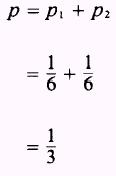EXAMPLE: From a bag containing 5 white balls, 2 black balls, and 11 red balls, 1 ball is drawn. What is the probability that it is either black or red? SOLUTION: The draw can be made in 18 ways. The choices are 2 black balls and 11 red balls, which are favorable, or a total of 13 favorable choices. Then, the probability of success is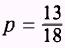Since drawing a red ball excludes the drawing of a black ball, and vice versa, the two events are mutually exclusive; so the probability of drawing a black ball is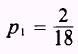and the probability of drawing a red ball is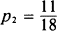Therefore, the probability of success isEXAMPLE: What is the probability of drawing either a king, a queen, or a jack from a deck of playing cards? SOLUTION: The individual probabilities areTherefore, the probability of success isEXAMPLE: What is the probability of rolling a die twice and having a 5 and then a 3 show or having a 2 and then a 4 show? SOLUTION: The probability of having a 5 and then a 3 show isand the probability of having a 2 and then a 4 show isThen, the probability of either p1 or p2 is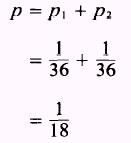PRACTICE PROBLEMS: When tossing a coin, you have what probability of getting either a head or a tail? 2. A bag contains 12 blue, 3 red, and 4 white marbles. What is the probability of drawing a. in 1 draw, either a red or a white marble? b. in 1 draw, either a red, white, or blue marble? c. in 2 draws, either a red marble followed by a blue marŁble or a red marble followed by a red marble? 3. What is the probability of getting a total of at least 10 points in rolling two dice? (HINT: You want either a total of 10, 11, or 12.) ANSWERS: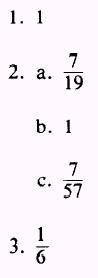Integrated Publishing, Inc. - A (SDVOSB) Service Disabled Veteran Owned Small Business